# Are ‘Possible Worlds’ Possible?

In recent decades, the idea in modal logic and metaphysics of possible worlds has become a widely used tool in philosophy. But are the hypotheticals discussed using possible worlds even, well, possible? To test this idea, I am going to try to construct an idea of possible worlds by way of mathematical models for making adjustments to the world as understood in both a metaphysically materialist/physicalist sense as well as what assumptions must be present for immaterialist/spiritualist claims to be true.

So, what is a possible world? A possible world is a state of affairs that could have obtained (i.e. it is not logically impossible, like a square circle) but it just so happened that it did not. I will quote here at length from the article on the Stanford Encyclopedia of Philosophy on what possible worlds are:

Anne is working at her desk. While she is directly aware only of her immediate situation — her being seated in front of her computer, the music playing in the background, the sound of her husband’s voice on the phone in the next room, and so on — she is quite certain that this situation is only part of a series of increasingly more inclusive, albeit less immediate, situations: the situation in her house as a whole, the one in her neighborhood, the city she lives in, the state, the North American continent, the Earth, the solar system, the galaxy, and so on. On the face of it, anyway, it seems quite reasonable to believe that this series has a limit, that is, that there is a maximally inclusive situation encompassing all others: things, as a whole or, more succinctly, the actual world.

Most of us also believe that things, as a whole, needn’t have been just as they are. Rather, things might have been different in countless ways, both trivial and profound. History, from the very beginning, could have unfolded quite other than it did in fact: the matter constituting a distant star might never have organized well enough to give light; species that survived might just as well have died off; battles won might have been lost; children born might never have been conceived and children never conceived might otherwise have been born. In any case, no matter how things had gone they would still have been part of a single, maximally inclusive, all-encompassing situation, a single world. Intuitively, then, the actual world of which Anne’s immediate situation is a part is only one among many possible worlds.

A version of the possible world is the subjunctive or counterfactual conditional. In a subjunctive counterfactual, it is stated that if some causal state of affairs had obtained, then some effect state of affairs would now obtain. An unstated assumption is that everything else in existence is held constant: the only things that are different are the cause and effect stated in the conditional. This unstated assumption, however, is not necessarily adequate. Not if, for instance, the counterfactual requires some change in the laws of physics in order for it to obtain.

As an example, we might say “if it had been the case that the dinosaurs never went extinct, then it would have been the case that humans would not have evolved.” However, this assumes either that causality does not occur in the way we think, or that the causal chain of events that led to the asteroid hitting the earth that caused the extinction of the dinosaurs would have to have been completely different. The former would require that some causal events that disobey causality as it is generally understood occurred, arbitrarily causing the asteroid to divert its path so as not to hit the earth. The latter assumes that, if we trace the world lines of each elementary particle in the actual universe, the counterfactual says that if those world lines had been different for the elementary particles involved in the asteroid impact, only then could the asteroid impact not have occurred. This, however, requires that the world lines for many different elementary particles be altered – indeed, all the ones within the light cone of the asteroid impact event, since those are all the elementary particles within causal range of one another.

That causality occurs differently than is understood is certainly possible (i.e. the laws of physics behave different than how we currently understand them; a providential deity intervenes in order to make certain things happen; or perhaps that certain causes, as we understand them, are only necessary, but not sufficient, for causality, and therefore other causal influences could take place). However, in order not to take these possibilities as an assumption, it either needs to be shown to be true, or be included as part of the subjunctive conditional. So, instead, we would say “if it had been the case that causality does not occur as it is generally understood (perhaps due to demonic influence) such that it is possible that the dinosaurs never went extinct, then it would have been the case that humans would not have evolved.”

Thus, we seem to have two different types of subjunctives here: those that state that the actual ontological structure of our universe is different in ways that would allow everything else to be held constant except for a single chain of events – namely, the asteroid missing earth and the subsequent course of evolution resulting in the preclusion of humanity – or that the world lines of all matter and energy involved in this subjunctive counterfactual, along with the resulting world lines of everything within the light cone of the counterfactual event, being altered so as to allow this event to occur.

### Ontologically Possible Worlds

We can think of all of the universe governing values (UGV) of the actual universe UA (i.e. physical constants such as the mass, charge, spin, chirality, etc. of every particle; the strength of the fundamental forces; the speed of light; the gravitational constant; the nature of Lagrangians, and other ontologically relevant values in the universe such as those facts/propositions about the universe that govern abstract objects, consciousness, and so on) as given by a column vector: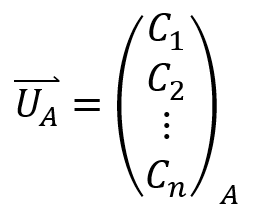Where each component is a different UGV. And so, in order to go from the actual universe  to the ontologically counterfactual universe UC, we can represent this as a change in the UGV’s as a transformation of  by the diagonal of a square n×n matrix H that I will call the ontological subjunctive matrix (OSM) so that:Where each matrix component given by μ = 1, 2, 3,…, n and ν = 1, 2, 3,…, n is:Where τ is the spacetime event being altered such that the change in a UGV is reflective of how much it would need to be altered in order to change the spacetime location of an object from the spacetime event τ in  to the spacetime event τ in UC. And so, changing the UGV’s of our actual universe can be represented by:If we want to stipulate a supernatural intervention in our possible universe, we need to show an arbitrary alteration in the relevant UGV’s for some period of time needed for the “miracle” to occur. For this we can use a time dependent change in the universe:Where D is a diagonal matrix with components being: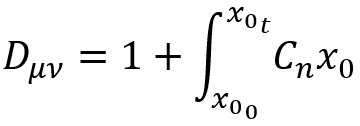When  is a function of time (the  dimension) and  is zero, then it reduces to the non-supernatural OSM. But, if the universe has some supernatural intervention between  and , then the integral will reflect this arbitrary change in whichever UGV’s are altered in order for the “miracle” to occur during that time period. The non-supernatural OSM, when matrix multiplied by the supernatural matrix, can give a single matrix which may be called the supernatural-allowable ontological subjunctive matrix or SOSM:And so we get:Where we can state the ontological subjunctive conditional as:What this fails to show, however, is whether changes in the UGV’s even allow for a viable universe (i.e. one that allows beings like humans to exist). It also doesn’t account for whether certain UGV’s could be added or subtracted (i.e. if we could have  or  different UGV’s in our possible universe). Presumably other UGV’s could be added or subtracted so as to alter some of the existing UGV’s without making the universe inviable. There is also the assumption here that all of the UGV’s are linearly independent, which may not be the case: it may be that altering any two or more independently leads to logical impossibilities. These are all considerations one would have to contemplate when positing a possible world.

### Causally Possible Worlds

A causally possible world is one where we posit some UGV’s and then use those values to determine what is physically allowable in our universe. Here, I will assume UGV’s of our actual world (or, at least, UGV’s that may be true of our actual world, depending on who one asks); I will also assume no supernatural intervention. If we think of the world line of some elementary particle n as a function that maps the probability density of location and momentum in phase space onto 4-dimensional Minkowski space:With q and p giving the positions and momenta in phase space such that the expectation value of an observable O:Is equal to 1 and where W, the Wigner quasiprobability distribution gives the phase space quasiprobability density by: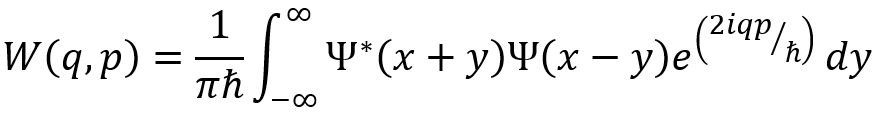And taking the Moyal bracket of  with the Hamiltonian such that the evolution of the Wigner quasiprobability distribution W in time isWhich I will define as:Where F(K) maps a particular point from the phase space evolution of a single particle onto the 4-dimensional spacetime manifold in Minkowski space. And so Ln = F(K) is a possible world line of a given elementary particle n as a function of its Wigner quasiprobability distribution W that evolves through time by the Moyal bracket with the Hamiltonian K = -{{W,H}}. Then we can give an n-dimensional vector representing a possible world line of each particle, where n is the number of elementary particles causally related to one another in one possible world that can be actualized given the evolutions of each particle’s Wigner quasiprobability distributions within the light cone of a given spacetime event τ:Where the lines in the actual world (where quantum effects exist) take each of these possible lines as components of a superposition: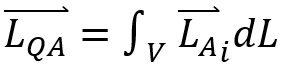With V being the volume of spacetime taken up by the group of infinitesimally narrow world lines (this could be further refined to take the probability amplitudes into effect using the path integral formalism, but for simplicity I am treating the bundle of world lines for a single particle like a narrow tube, composed of equally likely world lines, strung through spacetime, and neglecting paths that have only a minor probability outside this tube). And thus, a subjunctive counterfactual is represented as a square n×n matrix that I will call the causally subjunctive matrix (CSM). The CSM transforms the vector  composed of the functions whose values are the actual world lines into a vector  of counterfactual possible world lines. The CSM is: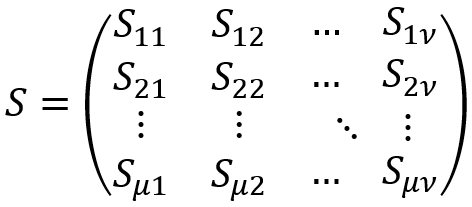With det(S) ≠ 0 and where each matrix component given by μ = 1, 2, 3,…, n and ν = 1, 2, 3,…, n is a differential operator: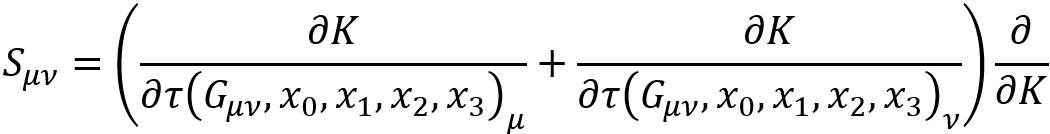With  the proper time as a function of spacetime and the Einstein tensor  (which is also a function of the spacetime and the energy density). And so, by manually altering the spacetime event τ (i.e. shifting objects in spacetime to suit the new state of affairs stipulated in the counterfactual) we end up with a possible world, represented by:And then we can state the subjunctive conditional as:We can then measure the proximity of a causally possible world in relation to the actual world:Where  is the average difference between the actual and counterfactual universes in all spacetime events over the entire universe and n is the number of particles in the universe. Thus, we can imagine an  differentiable manifold with the actual universe as the identity I and counterfactual universes being deviations from the identity generated by:This is an extremely simplified model that does not take into account whether the number of particles in the universe n is increased or decreased, the nature of abstract entities (i.e. it is purely reductionist), whether there are supernatural interventions and how much and how often, and the nature of consciousness and free will. Here I have assumed a clockwork universe with a constant n for simplicity.